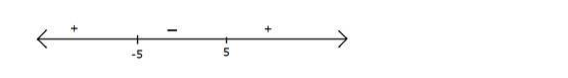# Solve

Question:

Solve $\frac{x}{x-5}>\frac{1}{2}$ when $x \in \mathbf{R}$.

Solution:

$\frac{x}{x-5}>\frac{1}{2}$

$\Rightarrow \frac{x}{x-5}-\frac{1}{2}>0$

$\Rightarrow \frac{2 x-x+5}{2(x-5)}>0$

$\Rightarrow \frac{x+5}{x-5}>0$

Observe that $\frac{x+5}{x-5}$ is zero at $\mathrm{x}=-5$ and not defined at $\mathrm{x}=5$

Hence plotting these two points on number line

Now for $x>5, \frac{x+5}{x-5}$ is positive

For every root and not defined value of $\frac{x+5}{x-5}$ the sign will changeWe want greater than zero that is the positive part hence x < -5 and x > 5

$x<-5$ means $x$ is from negative infinity to $-5$ and $x>5$ means $x$ is from 5 to infinity

Hence $x \in(-\infty,-5) \cup(5, \infty)$

Hence solution of $\frac{x}{x-5}>\frac{1}{2}$ is $x \in(-\infty, 2) \cup(5, \infty)$